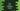# C++ program to print Fibonacci series## C++ program to print Fibonacci series:

In this post, we will learn how to print a fibonacci sequence in C++. A Fibonacci sequence is a sequence where each item is equal to sum of the previous two items. The first two items of this series are 0 and 1.

It looks like 0, 1, 1, 2, 3, 5, 8, 13, 21….

In our program, we will take the total count of numbers to show. Based on that, the program will print the Fibonacci series.

### C++ program:

Below is the complete C++ program:

``````#include <iostream>

using namespace std;

int main()
{
int total, first = 0, second = 1, sum;

cout << "Enter total numbers to show : "<<endl;
cin>>total;

for (int i = 0; i < total; i++)
{
if (i == 0)
{
cout << first << " ";
}
else if (i == 1)
{
cout << second << " ";
}
else
{
sum = first + second;
cout << sum << " ";
first = second;
second = sum;
}
}
}``````

### Explanation:

In this program,

• total is used to save the total numbers to show in the series. This value is entered by the user.
• first and second are two integer variables to store the first and the second values of the series i.e. 0 and 1.
• The for loop runs from i = 0 to i = total - 1. For the first and the second values, it prints first and second. For other values, it will print first + second. Also, it updates the values of first and second by assigning the value of second to first and sum to second.

### Output:

This program will print output as like below:

``````Enter total numbers to show :
1
0

Enter total numbers to show :
2
0 1

Enter total numbers to show :
5
0 1 1 2 3

Enter total numbers to show :
20
0 1 1 2 3 5 8 13 21 34 55 89 144 233 377 610 987 1597 2584 4181``````